2019-11-05 00:07:52 qq_36511401 阅读数 7571
• ###### 从工程角度再探《线性代数》

从工程角度探讨线代的典型应用，通过“刷实例”来培养解决工程问题的数学直觉。 课程安排 1.从新的角度讲解线性代数中最重要的知识点；(从有别于大学课程的角度，更深入理解线性代数)       向量、矩阵、矩阵乘法、方程组、矩阵分解... 2.探讨每一个知识点的典型应用；      推荐系统中的相似性度量     图像处理中的低通滤波及边缘检测     游戏中的图形变换      天文学中的轨道参数估计     数据降维中的主成分分析     人脸识别     搜索引擎中的网页排名     基于协同过滤的推荐系统 3.用Python实现上述应用。 总结起来，就是每学完一个知识点，就给出相关实例，并用Python语言做对应的代码实践！

467 人正在学习 去看看 刘高联

1、选择一张椒盐噪声比较明显的图片。椒盐噪声，椒盐噪声又称脉冲噪声，它随机改变一些像素值，是由图像传感器，传输信道，解码处理等产生的黑白相间的亮暗点噪声。椒盐噪声往往由图像切割引起。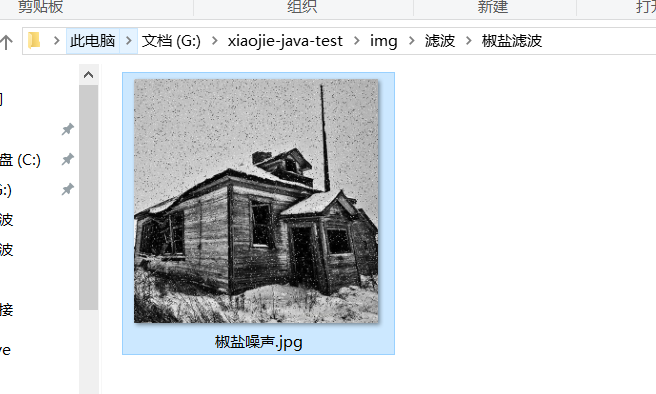2、代码。生成模版半径分别是3、5和7的图片。

``````package com.zxj.reptile.test.mnist;

import com.zxj.reptile.utils.image.ImageService;
import com.zxj.reptile.utils.image.ImageUtils;

public class ImgTest {

private static final String File_Path = "G:\\xiaojie-java-test\\img\\";

public static void main(String[] args) {
//中值滤波
String gaussSourcePath = File_Path + "滤波\\椒盐滤波\\椒盐噪声.jpg";
String averageTargetPath = File_Path + "滤波\\椒盐滤波\\椒盐滤波_";
ImageService.middleFilter(gaussSourcePath, averageTargetPath, ImageUtils.Gray_Type_Default, 3);//中值过滤
ImageService.middleFilter(gaussSourcePath, averageTargetPath, ImageUtils.Gray_Type_Default, 5);//中值过滤
ImageService.middleFilter(gaussSourcePath, averageTargetPath, ImageUtils.Gray_Type_Default, 7);//中值过滤
}
}``````
``````package com.zxj.reptile.utils.image;

import javax.imageio.ImageIO;
import java.awt.image.BufferedImage;
import java.io.File;

public class ImageService {
/**
* 中值过滤
*
* @param sourcePath   原图像
* @param targetPath   目标图像
* @param grayType     灰度化方式
* @param filterLength 过滤的长度（阈值）
*/
public static void middleFilter(String sourcePath, String targetPath, int grayType, int filterLength) {
try {
//获取原图像对象，并获取原图像的二维数组
int[][] imgArrays = ImageUtils.getTwoDimension(image);
//生成新图像的二维数组
imgArrays = ImageUtils.getGrayImg(imgArrays, grayType);//灰度化
int[][] newImgArrays = ImageUtils.getMiddleFilter(imgArrays, filterLength);//中值过滤
//生成新图片对象，填充像素
BufferedImage newImage = new BufferedImage(image.getWidth(), image.getHeight(), BufferedImage.TYPE_BYTE_GRAY);
ImageUtils.setTwoDimension(newImage, newImgArrays, ImageUtils.Channel_Type_1);
//生成图片文件
ImageIO.write(newImage, "JPEG", new File(targetPath + filterLength + ".jpg"));
} catch (Exception e) {
e.printStackTrace();
}
}
}
``````
``````package com.zxj.reptile.utils.image;

import com.zxj.reptile.utils.algorithm.SortUtils;

import java.awt.image.BufferedImage;

public class ImageUtils {
/**
* 中值滤波
*
* @param imgArrays    图像二维数组
* @param filterLength 过滤的长度（阈值）
*/
public static int[][] getMiddleFilter(int[][] imgArrays, int filterLength) {
final int imgHeight = imgArrays.length;
final int imgWidth = imgArrays.length;
int[][] newImgArrays = new int[imgHeight][imgWidth];
//模版半径
final int filterRadius = (filterLength - 1) / 2;
final int filterSize = filterLength * filterLength;
//获取数据
for (int h = 0; h < imgHeight; h++) {//图片第几行
for (int w = 0; w < imgWidth; w++) {//图片第几列
int count = 0;
int[] templateArray = new int[filterSize];
int rowIndex = h + templateH;
if (rowIndex < 0 || rowIndex > imgHeight - 1) {
continue;
}
int columnIndex = w + templateW;
if (columnIndex < 0 || columnIndex > imgWidth - 1) {
continue;
}
templateArray[count++] = imgArrays[rowIndex][columnIndex];
}
}
//
int[] newTemplateArray;
if (count != templateArray.length) {
newTemplateArray = new int[count];
for (int i = 0; i < count; i++) {
newTemplateArray[i] = templateArray[i];
}
SortUtils.countSort(newTemplateArray);//计数排序
newImgArrays[h][w] = newTemplateArray[count / 2];
} else {
SortUtils.countSort(templateArray);//计数排序
}
}
}
return newImgArrays;
}

//灰度处理的方法
public static final byte Gray_Type_Default = 0;//默认加权法
public static final byte Gray_Type_Min = 1;//最大值法
public static final byte Gray_Type_Max = 2;//最小值法
public static final byte Gray_Type_Average = 3;//平均值法
public static final byte Gray_Type_Weight = 4;//加权法
public static final byte Gray_Type_Red = 5;//红色值法
public static final byte Gray_Type_Green = 6;//绿色值法
public static final byte Gray_Type_Blue = 7;//蓝色值法

//生成的图片是几通道的
public static final byte Channel_Type_Default = 0;//默认三通道
public static final byte Channel_Type_1 = 1;//单通道
public static final byte Channel_Type_3 = 3;//三通道

/**
* 灰度化处理
*
* @param imgArrays 图像二维数组
* @param grayType  灰度化方法
*/
public static int[][] getGrayImg(int[][] imgArrays, int grayType) throws Exception {
final int imgHeight = imgArrays.length;
final int imgWidth = imgArrays.length;
int[][] newImgArrays = new int[imgHeight][imgWidth];
for (int h = 0; h < imgHeight; h++) {
for (int w = 0; w < imgWidth; w++) {
final int[] grb = getRgb(imgArrays[h][w]);
newImgArrays[h][w] = getGray(grb, grayType);
}
}
return newImgArrays;
}

/**
* 通过像素值，返回r、g、b颜色通道的值
*
* @param pixel 像素值
*/
public static int[] getRgb(int pixel) {
int[] rgb = new int;
rgb = (pixel >> 16) & 0xff;
rgb = (pixel >> 8) & 0xff;
rgb = pixel & 0xff;
return rgb;
}

/**
* 根据不同的灰度化方法，返回灰度值
*
* @param rgb      r、g、b颜色通道的值
* @param grayType 不同灰度处理的方法
*/
public static int getGray(int[] rgb, int grayType) throws Exception {
if (grayType == Gray_Type_Average) {
return (rgb + rgb + rgb) / 3;   //rgb之和除以3
} else if (grayType == Gray_Type_Weight || grayType == Gray_Type_Default) {
return (int) (0.3 * rgb + 0.59 * rgb + 0.11 * rgb);
} else if (grayType == Gray_Type_Red) {
return rgb;//取红色值
} else if (grayType == Gray_Type_Green) {
return rgb;//取绿色值
} else if (grayType == Gray_Type_Blue) {
return rgb;//取蓝色值
}
//比较三个数的大小
int gray = rgb;
for (int i = 1; i < rgb.length; i++) {
if (grayType == Gray_Type_Min) {
if (gray > rgb[i]) {
gray = rgb[i];//取最小值
}
} else if (grayType == Gray_Type_Max) {
if (gray < rgb[i]) {
gray = rgb[i];//取最大值
}
} else {
throw new Exception("grayType出错");
}
}
return gray;
}

/**
* 获取二维像素
*
* @param image BufferedImage图像对象
*/
public static int[][] getTwoDimension(BufferedImage image) {
final int imgWidth = image.getWidth();
final int imgHeight = image.getHeight();
int[][] imgArrays = new int[imgHeight][imgWidth];
for (int i = 0; i < imgHeight; i++) {
for (int j = 0; j < imgWidth; j++) {
imgArrays[i][j] = image.getRGB(j, i);
}
}
return imgArrays;
}

/**
* 将二维像素填充到图像中
*
* @param image       BufferedImage图像对象
* @param imgArrays   二维像素
* @param channelType 单通道还是三通道
*/
public static void setTwoDimension(BufferedImage image, int[][] imgArrays, int channelType) throws Exception {
final int imgWidth = image.getWidth();
final int imgHeight = image.getHeight();
for (int i = 0; i < imgHeight; i++) {
for (int j = 0; j < imgWidth; j++) {
if (channelType == Channel_Type_1) {
image.setRGB(j, i, (byte) imgArrays[i][j]);
} else if (channelType == Channel_Type_3 || channelType == Channel_Type_Default) {
image.setRGB(j, i, imgArrays[i][j]);
} else {
throw new Exception("channelType错误");
}
}
}
}
}
``````

3、结果。半径越长，图像越模糊，效果越不理想。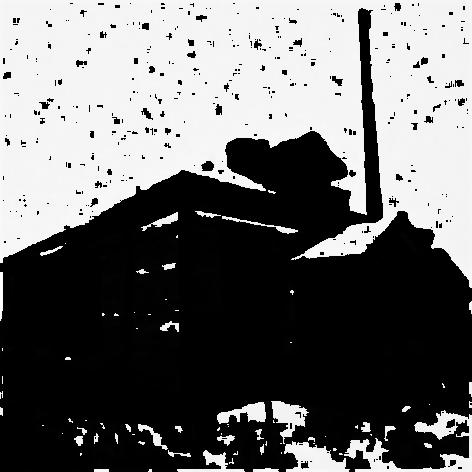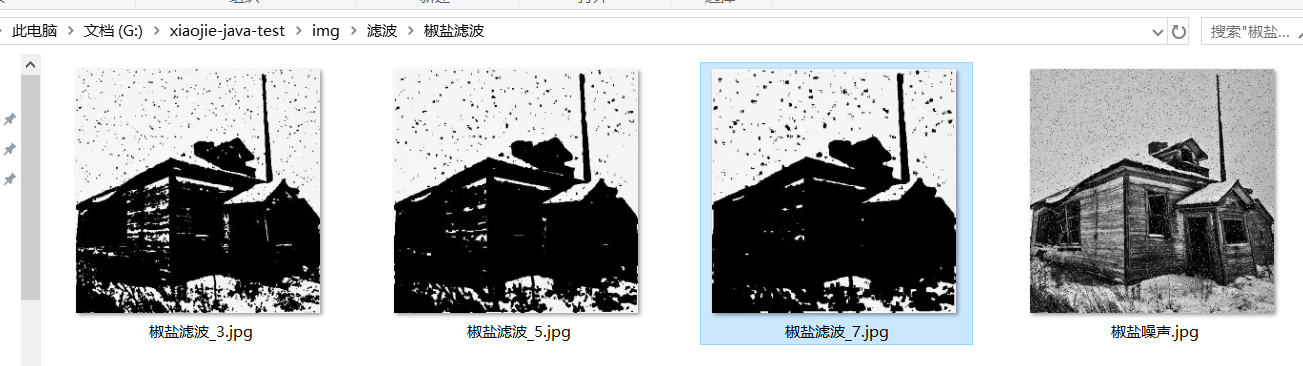4、结论。

中值滤波采用非线性的方法，它在平滑脉冲噪声方面非常有效,同时它可以保护图像尖锐的边缘，选择适当的点来替代污染点的值，所以处理效果好，对椒盐噪声表现较好，对高斯噪声表现较差。半径越长，图像越模糊，效果越不理想。

2019-02-21 21:07:45 qq_34725005 阅读数 304
• ###### 从工程角度再探《线性代数》

从工程角度探讨线代的典型应用，通过“刷实例”来培养解决工程问题的数学直觉。 课程安排 1.从新的角度讲解线性代数中最重要的知识点；(从有别于大学课程的角度，更深入理解线性代数)       向量、矩阵、矩阵乘法、方程组、矩阵分解... 2.探讨每一个知识点的典型应用；      推荐系统中的相似性度量     图像处理中的低通滤波及边缘检测     游戏中的图形变换      天文学中的轨道参数估计     数据降维中的主成分分析     人脸识别     搜索引擎中的网页排名     基于协同过滤的推荐系统 3.用Python实现上述应用。 总结起来，就是每学完一个知识点，就给出相关实例，并用Python语言做对应的代码实践！

467 人正在学习 去看看 刘高联

``````import numpy as np
import scipy.ndimage
import cv2

def convert_2d(r):
n = 10
s = scipy.ndimage.median_filter(r, (n, n))
return s.astype(np.uint8)

def convert_3d(r):
s_dsplit = []
for d in range(r.shape):
rr = r[:, :, d]
ss = convert_2d(rr)
s_dsplit.append(ss)
s = np.dstack(s_dsplit)
return s

im_convert_mat = convert_3d(im)
cv2.imshow('median', im_convert_mat)
cv2.imwrite('median.jpg', im_convert_mat)
cv2.waitKey()
``````2015-06-09 20:29:55 lvlitc 阅读数 6417
• ###### 从工程角度再探《线性代数》

从工程角度探讨线代的典型应用，通过“刷实例”来培养解决工程问题的数学直觉。 课程安排 1.从新的角度讲解线性代数中最重要的知识点；(从有别于大学课程的角度，更深入理解线性代数)       向量、矩阵、矩阵乘法、方程组、矩阵分解... 2.探讨每一个知识点的典型应用；      推荐系统中的相似性度量     图像处理中的低通滤波及边缘检测     游戏中的图形变换      天文学中的轨道参数估计     数据降维中的主成分分析     人脸识别     搜索引擎中的网页排名     基于协同过滤的推荐系统 3.用Python实现上述应用。 总结起来，就是每学完一个知识点，就给出相关实例，并用Python语言做对应的代码实践！

467 人正在学习 去看看 刘高联

图像滤波大多数用于图像的模糊处理和减小噪声（个人理解）。常见的滤波有：均值滤波，中值滤波，高斯滤波等。在这我主要介绍一下中值滤波，它对于处理椒盐噪声十分有效。下面我简单介绍下它的原理，以及我自己写的中值滤波函数。

均值滤波：

对一副图像上的某个点进行中值滤波处理，先将掩模内欲求的像素及其邻域的像素值排序，确定出中值，然后将这个中值赋值给该像素。如图所示一个5 x 5邻域中，用中值124代替中心的像素值。```Mat MedianFilter(Mat img) //中值滤波器
{
int count = 0;   //领域中像素个数
int index=0;
int pixel1;     //数组存放像素值
int pixel2;
int pixel3;
for (int x = 1; x < img.rows-1; x++)   //遍历像素，并用邻域像素的中值代替该像素
{
for (int y = 1; y < img.cols-1; y++)
{
count = 0;
for (int row = -kernel_size / 2; row <= kernel_size / 2; row++)  //计算邻域像素
{
int row2 = x + row;
for (int col = -kernel_size / 2; col <= kernel_size / 2; col++)
{
int col2 = y + col;
pixel1[count] = *(img.data + img.step * row2 + img.step * col2);  //将邻域像素放入数组
pixel2[count] = *(img.data + img.step * row2 + img.step * col2 + img.elemSize1());
pixel3[count] = *(img.data + img.step * row2 + img.step * col2 + img.elemSize1()*2);
count++;
}
}
bubblingsort(pixel1,count);  //排序
bubblingsort(pixel2, count);
bubblingsort(pixel3, count);
*(img.data + img.step * x + img.step * y) = pixel1[count/2];  //用邻域像素的中值代替该像素
*(img.data + img.step * x + img.step * y + img.elemSize1()) = pixel2[count/2];
*(img.data + img.step * x + img.step * y + img.elemSize1()*2) = pixel3[count / 2];
}

}
return img;
}```

注：我的代码中没有对图像的边缘像素进行处理，由于输入输出是同一张图，所以边缘像素依旧为原来的像素。
运行结果：
原图（带椒盐噪声）：                                                                                                     处理后（中值滤波）：显然中值滤波对去除椒盐噪声有着明显的作用，但由于能力有限，并没有完全达到去除的效果，而且感觉有部分细节丢失，欢迎大神随时指点补充交流！

转载请说明出处！谢谢

2019-09-14 16:56:03 weixin_44225182 阅读数 1992
• ###### 从工程角度再探《线性代数》

从工程角度探讨线代的典型应用，通过“刷实例”来培养解决工程问题的数学直觉。 课程安排 1.从新的角度讲解线性代数中最重要的知识点；(从有别于大学课程的角度，更深入理解线性代数)       向量、矩阵、矩阵乘法、方程组、矩阵分解... 2.探讨每一个知识点的典型应用；      推荐系统中的相似性度量     图像处理中的低通滤波及边缘检测     游戏中的图形变换      天文学中的轨道参数估计     数据降维中的主成分分析     人脸识别     搜索引擎中的网页排名     基于协同过滤的推荐系统 3.用Python实现上述应用。 总结起来，就是每学完一个知识点，就给出相关实例，并用Python语言做对应的代码实践！

467 人正在学习 去看看 刘高联

## 中值滤波

概念
中值滤波是基于排序统计理论的一种能有效抑制噪声的非线性信号处理技术，中值滤波的基本原理是把数字图像或数字序列中一点的值用该点的一个邻域中各点值的中值代替，让周围的像素值接近的真实值，从而消除孤立的噪声点。方法是用某种结构的二维滑动模板，将板内像素按照像素值的大小进行排序，生成单调上升（或下降）的为二维数据序列。二维中值滤波输出为g（x,y）=med{f(x-k,y-l),(k,l∈W)} ，其中，f(x,y)，g(x,y)分别为原始图像和处理后图像。W为二维模板，通常为33，55区域，也可以是不同的的形状，如线状，圆形，十字形，圆环形等。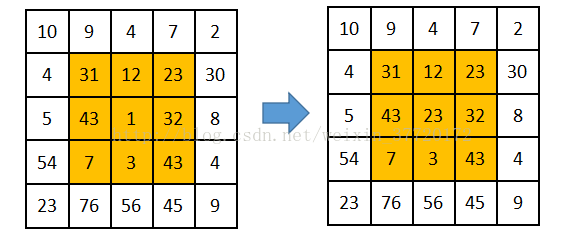## MATLAB实现

`````` t=imread('a1.jpg');
t=rgb2gray(t);
t1=imnoise(t,'salt & pepper',0.3);
subplot(1,2,1),imshow(t1),title('加入椒盐噪声后')
t2=medfilt2(t1,[3 3]);
subplot(1,2,2),imshow(t2),title('中值滤波后')
``````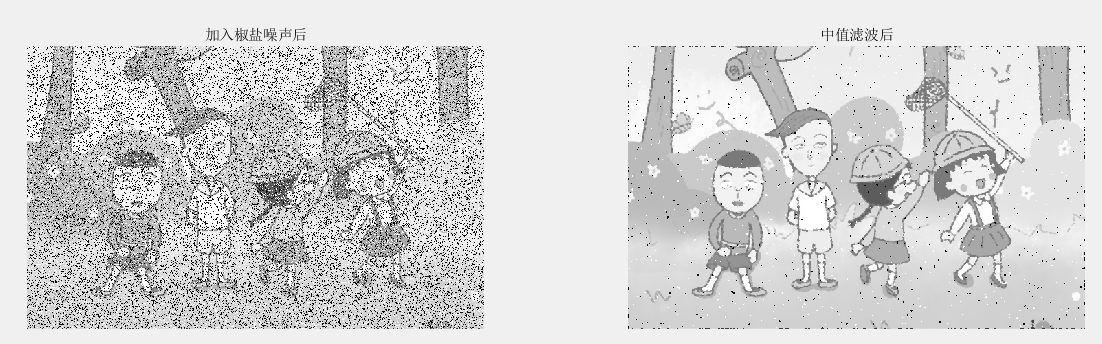``````t=imread('a1.jpg');
t1=imnoise(t,'salt & pepper',0.3);
subplot(1,2,1),imshow(t1),title('加入椒盐噪声后')
t2=t
t2(:,:,1)=medfilt2(t1(:,:,1),[3 3]);
t2(:,:,2)=medfilt2(t1(:,:,2),[3 3]);
t2(:,:,3)=medfilt2(t1(:,:,3),[3 3]);
subplot(1,2,2),imshow(t2),title('中值滤波后')
````````````function [ img ] = median_filter( image, m )
%----------------------------------------------
%中值滤波
%输入：
%image：原图
%m：模板的大小3*3的模板，m=3

%输出：
%img：中值滤波处理后的图像
%----------------------------------------------
n = m;
[ height, width ] = size(image);
x1 = double(image);
x2 = x1;
for i = 1: height-n+1
for j = 1:width-n+1
mb = x1( i:(i+n-1),  j:(j+n-1) );%获取图像中n*n的矩阵
mb = mb(:);%将mb变成向量化，变成一个列向量
mm = median(mb);%取中间值
x2( i+(n-1)/2,  j+(n-1)/2 ) = mm;

end
end

img = uint8(x2);
end
``````

``````t=imread('a1.jpg');
t1=imnoise(t,'salt & pepper',0.3);
imshow(t1),title('加入椒盐噪声');
t2=median_filter(t,3);%调用函数
figure,imshow(t),title('中值滤波后')
``````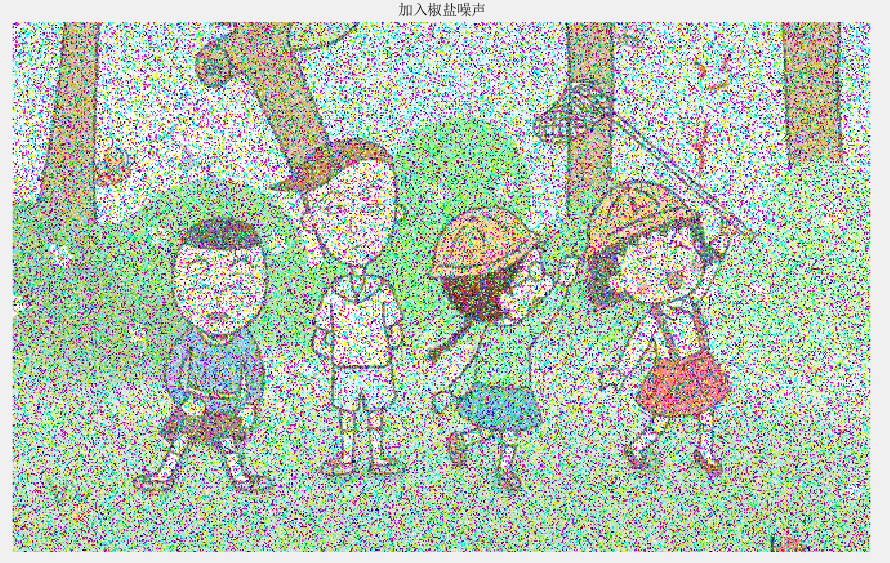## 更多

2013-07-11 17:54:37 saintfox001 阅读数 3951
• ###### 从工程角度再探《线性代数》

从工程角度探讨线代的典型应用，通过“刷实例”来培养解决工程问题的数学直觉。 课程安排 1.从新的角度讲解线性代数中最重要的知识点；(从有别于大学课程的角度，更深入理解线性代数)       向量、矩阵、矩阵乘法、方程组、矩阵分解... 2.探讨每一个知识点的典型应用；      推荐系统中的相似性度量     图像处理中的低通滤波及边缘检测     游戏中的图形变换      天文学中的轨道参数估计     数据降维中的主成分分析     人脸识别     搜索引擎中的网页排名     基于协同过滤的推荐系统 3.用Python实现上述应用。 总结起来，就是每学完一个知识点，就给出相关实例，并用Python语言做对应的代码实践！

467 人正在学习 去看看 刘高联

——（完整工程文件到我的资源下载）

1.图像中值滤波的背景和意义

2.中值滤波的原理方法

n为偶数时，y=x(k1)+x(k2),k1=n/2,k2=k1+1。

3*3的模板，对9个数排序，取第5个数替代原来的像素值。以某一个像素点为中心，选取一个3*3的窗口为模版，如图11-2-3：模版中的灰度值依次为｛2,1,4,2,2,3,7,6,8｝，排序后新的灰度值为｛1,2,2,2,3,4,6,7,8｝,第五个数3为排序后的中值，用3取代原来的中心值2，完成中值滤波过程。1选择一个(2n+1)×(2n+1)的窗口(通常为3×3或5×5)，并用该窗口沿图像数据进行行或列方向的移位滑动；

2 每次移动后，对窗内的诸像素灰度值进行排序；

3 用排序所得中值替代窗口中心位置的原始像素灰度值。

3.实验的C语言实现代码

/*========  头文件引用===========*/

#include "stdio.h"

#include "math.h"

/*============= 工作变量定义======*/

unsigned char *pr_n;    //指针定义

unsigned char *pr_s;    //指针定义

//说明：定义数据存放变量

#pragma        DATA_SECTION(IMG,"data");

int  IMG;

#pragma        DATA_SECTION(Noise_IMG,"data");

unsigned char  Noise_IMG;

#pragma        DATA_SECTION(Smooth_IMG,"data");

unsigned char  Smooth_IMG;

void  Medel_Smooth();

///////////////////////////////////////////////////////////////////////////////////////////////////////////////

//使用说明：

//   1. 本程序可以在Simulator下运动;

//   2. 程序编译、链接、加载成功后，先

//     （说明：*.dat格式，内部存放了某图像各像素的灰度值）加载入数据存储器存储地址Noise_IMG中

//   3. 数据加载成功后，再Debug/Go Main, 一步一步运行程序

///////////////////////////////////////////////////////////////////////////////////////////////////////////////

/*================= 主程序 ================*/

main()

{

long n;

int imgH,imgW;

int *ptr;

imgH=160;  //图像高与宽，因为数据文件中的图像是160X160像素的

imgW=160;

/*=========== 初始化 ==========*/

//1 把图像数据从IMG中移到Noise_IMG数组中

ptr=IMG;

pr_n=Noise_IMG;

for (n=0;n<imgH*imgW;n++)

*pr_n++=*ptr++;

//说明：在此暂停，可看到噪声图像

//指针指向数组

pr_n=Noise_IMG;

pr_s=Smooth_IMG;

//2 调用子程序，进行彩色图像变换成灰度图像

while (1)

{

Medel_Smooth(pr_n,pr_s,imgW,imgH);

//说明：上面子程序执行后，在此暂停，可看平滑后的图像

}

//说明：在此暂停，可看变换后的灰度图像

}

/*============== 子程序 =============*/

void Medel_Smooth

(   unsigned char *sourceImg,     //原始图像

unsigned char *newImg,       //输出：中值滤波后图像

int cols, int rows           //图像的宽度与高度     /* 图像的宽度与高度            */

)

{     //定义局部变量

int i,j,k,m,r,range=32;

int pixels;

unsigned char *pp=sourceImg, *newpp=newImg;

int medianWin={0};

int temp;

for (i=0; i< cols -1; i++)

*newpp++ = *pp++;

pp  =  sourceImg + (rows-1)* cols;

newpp =  newImg+ (rows-1)* cols;

for (i=0; i< cols -1; i++)

*newpp++ = *pp++;

newpp  =  newImg;

pp     =  sourceImg;

for (i=0; i< rows -1; i++)

{           *newpp = *pp;

newpp+=cols; pp+=cols;

}

newpp  =  newImg+cols-1;

pp     =  sourceImg+cols-1;

for (i=0; i< rows -1; i++)

{

*newpp = *pp;

newpp+=cols; pp+=cols;

}

pixels=(cols-2)*(rows-2);

pp=sourceImg+cols+1;

newpp=newImg+cols+1;

for(i=0;i<pixels;i++)

{

medianWin=*(pp - cols-1);

medianWin=*(pp -cols);

medianWin=*(pp - cols+1);

medianWin=*(pp-1);

medianWin=*pp;

medianWin=*(pp+1);

medianWin=*(pp + cols-1);

medianWin=*(pp + cols);

medianWin=*(pp + cols+1);

r=8;

for(k=0;k<r;k++,r--)

for(m=0;m<r;m++)

{

{if ( medianWin[m]>medianWin[m+1] )

{temp=medianWin[m];

medianWin[m]=medianWin[m+1];

medianWin[m+1]=temp;

}

}

}

*newpp=medianWin; //排序后取中间值赋值*newpp

newpp++;

pp++; }            }//程序结束

4.实验步骤与结果

1实验设备

1. PC兼容机一台：操作系统为Windows XP (Windows NT、Windows 98、Windows 2000)，Windows操作系统的内核如果是NT的英安装相应的补丁程序(如：Windows 2000为Service Pack3，Windows XP为 Service Pack1)。

2. Code Composer Studio 2.2 软件开发环境。

3. TS-DM64x实验箱一台（软件仿真可以不要实验箱）。

2实验步骤

1设置ccs为软件仿真环境2. 启动CCS。

3. 打开工程，编译并运行程序。

4. 运行程序，观察试验结果。

（1）编译、链接程序：执行菜单Project/Rebuild All，汇编结果在将汇编信息输出窗口中给出。编译后将在Bebug目录中产生一个ImgSmooth.out文件。（4）运行程序：执行Debug/Run。为了便于观看试验前后的结果，可以在程序中设置断点，采用单步执行的方法运行程序。

（5）显示平滑前的噪声图像：执行View/Graph/Image，在弹出的对话框中选择颜色类型为RGB，并输入RGB彩色图像三个通道数据的地址，以及图像显示格式（显示几行、每行几像素）等内容，如下图所示。这样，原始的噪声图像如图1所示。

（6）显示平滑后的图像：执行View/Graph/Image，在弹出的对话框中选择颜色类型为RGB，并输入灰度图像据的地址，以及图像显示格式（显示几行、每行几像素）等内容，如下图所示。这样平滑后的图像如图2(7)按照上述方法加载带有椒盐噪声的图像，并进行中值滤波，观察实验结果。如3图所示5.实验结果分析与总结